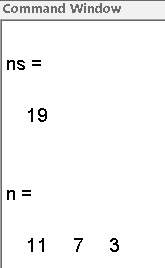• 席位分配的优化模型.pdf席位分配的优化模型.pdf席位分配的优化模型.pdf席位分配的优化模型.pdf席位分配的优化模型.pdf席位分配的优化模型.pdf
• ## 公平的席位分配

千次阅读 2016-02-18 18:53:16
“公平”的席位分配首先本来就是不可能的，公平一般是无法达到的，我们只是尽量降低不公平度，那么我们怎么衡量不公平度呢。就像评价一个人，有不同的指标，不公平度也是一样，这里介绍一种相对合理易于接受，且好...
如果说数学有点用，估计大都表现在运筹学中吧。“公平”的席位分配首先本来就是不可能的，公平一般是无法达到的，我们只是尽量降低不公平度，那么我们怎么衡量不公平度呢。就像评价一个人，有不同的指标，不公平度也是一样，这里介绍一种相对合理易于接受，且好判断的方法。

问题表述

某学校三个系部学生共200名,(甲系100,乙系60,丙系40)代表会议共20席,按比例分配三个系分别为10、6、4席。老情况变为下列情况怎样分配才是最公平的,现因学生转系三系人数为103、63、34。
1. 问20席该如何分配 ?
2. 若增加21席又如何分配 ?

显然，因为无法整除无论如何分配都不公平。下面说一下几种策略。
1. 按班级人数比例乘以总人数，小数点大的分得多余的一个位子。

某校
甲系
乙系
丙系

共200人
103
63
34

人数比例
51.3
31.5
17

20席位
10.3
6.3
3.4

实际分配
10
6
4

21席位
10.82
6.62
3.57

实际分配
11
7
3

按照上述方法，会出现席位增多而丙系的席位却减少了一个的不合理现象，说明此方法并不合理。

模型建立

假设由A、B两个单位公平分配席位的情况，设两方人数p1,p2$p_1,p_2$ ,分配到的席位为 n1,n2$n_1,n_2$。
1. p1n1=p2n2$\frac{p_1}{n_1} = \frac{p_2}{n_2}$ 公平，但是一般是不满足的。
2. p1n1>p2n2$\frac{p_1}{n_1} > \frac{p_2}{n_2}$ 对A不公平。
3. p1n1<p2n2$\frac{p_1}{n_1} < \frac{p_2}{n_2}$ 对B不公平。

绝对不公平度为
d=∣∣∣p1n1−p2n2∣∣∣ d = \left| \frac{p_1}{n_1} - \frac{p_2}{n_2} \right|
但这样做还是有不足，例如
某两个单位的人数和席位为 n1=n2=10,p1=120,p2=100$n_1=n_2=10,p_1=120,p_2=100$ 算得  d=2$d=2$.
另两个单位的人数和席位为 n1=n2=10,p1=1020,p2=1000$n_1=n_2=10,p_1=1020,p_2=1000$ 算得 d=2$d=2$。
但是显然，后一种方案，更公平。

因此，我们引入相对不公平度
1. 若 p1n1>p2n2$\frac{p_1}{n_1} > \frac{p_2}{n_2}$ 则
rA(n1,n2)=p1n1−p2n2p2n2 r_A(n_1,n_2) = \frac{ \frac{p_1}{n_1} - \frac{p_2}{n_2} }{ \frac{p_2}{n_2} }
2. 若 p1n1<p2n2$\frac{p_1}{n_1} < \frac{p_2}{n_2}$
rB(n1,n2)=p2n2−p1n1p1n1 r_B(n_1,n_2) =  \frac{\frac{p_2}{n_2} - \frac{p_1}{n_1} }{\frac{p_1}{n_1}}
我们的目标是让rA,rB$r_A,r_B$(每种分配只会有一个)最小。

确定分配方案

假设当前 p1n1>p2n2$\frac{p_1}{n_1} > \frac{p_2}{n_2}$ 对A不公平。新增了一个席位。
1. 若 p1n1+1>p2n2$\frac{p_1}{n_1+1} > \frac{p_2}{n_2}$ 则A加1席
2. 若 p1n1+1<p2n2$\frac{p_1}{n_1+1} < \frac{p_2}{n_2}$
若分配给A，则对B的不公平值(相对):
rB(n1+1,n2)=p2(n1+1)p1n2−1 r_B(n_1+1,n_2) = \frac{p_2(n_1+1)}{p_1 n_2} -1
若分配给B，则对A的不公平值(相对):
rA(n1,n2+1)=p1(n2+1)p2n1−1 r_A(n_1,n_2+1) = \frac{p_1(n_2+1)}{p_2 n_1} -1

若rA(n1,n2+1)<rB(n1+1,n2)$r_A(n_1,n_2+1)则席位分配给B，反之给A。
即令
Qi=p2ini(ni+1) Q_i = \frac{p_i ^2}{n_i(n_i+1)}
将席位分配给 Q$Q$ 值较大者。

模型求解

先按照平均原则取整之后。分出了19席：n1=10,n2=6,n3=3$n_1=10,n_2=6,n_3=3$
第20席:
Q1=103210×11=96.4,Q2=6326×7=94.5,Q3=3423×4=96.3 Q_1 = \frac{103^2}{10 \times 11 } = 96.4 \; , \;Q_2 = \frac{63^2}{6 \times 7} = 94.5 \; , \;Q_3 = \frac{34^2}{3 \times 4} = 96.3
则分配：n1=11,n2=6,n3=3$n_1=11,n_2=6,n_3=3$
第21席：Q1=80.4,Q2=94.5,Q3=96.3$Q_1=80.4, Q_2 = 94.5, Q_3 = 96.3$
则分配：n1=11,n2=6,n3=4$n_1=11,n_2=6,n_3=4$.

本文参考文库1和文库2。修改了其中的错误，由于席位分配问题确实是一个经典问题，故在此记录。

欢迎访问http://dna049.com
展开全文数学
• Q值法是20世纪20年代哈佛大学数学家E.V.Huntington提出和推荐的席位分配算法中的一个方法 下面对这类Huntington除法做出简单介绍和比较 设共有m方分配N个席位，第i方人数为pi,记p = (p1,p2,p3…pm),P = Σ（i从1到...
Q值法：增加的一个席位时，计算Qi=pi^2/[ni*(ni+1)],I = 1,2,3… 增加的一个席位应当分配给Q值最大的一方
Q值法是20世纪20年代哈佛大学数学家E.V.Huntington提出和推荐的席位分配算法中的一个方法
下面对这类Huntington除法做出简单介绍和比较
设共有m方分配N个席位，第i方人数为pi,记p = (p1,p2,p3…pm),P = Σ（i从1到m）Pi.席位分配要求
寻找n=(n1,n2,n3…nm),其中ni是第i方分配的席位，满足Σ（i从1到m）ni=N,且ni均为非负整数
按照最大剩余法（GR）分配席位时，首先计算第i方精确的席位份额，记为qi,可得到qi=N*pi/P, i=1,2,3…m.
若qi均为整数，令ni=qi即可完成分配。否则记[qi]=qi的整数部分，先将[qi]分给第i方，然后将尚未分配的
r - Σ（i从1到m）[qi]个席位分配给剩余qi-[qi]中最大的r方，每方一席
对上述方法进行思考：
1.进行席位分配最终结果肯定是所有的席位都已经分配出去了。这句对应的就是上面的满足Σ（i从1到m）ni=N
2.由于分配时不一定按照份额分配都能得到整数，因此绝大多数的情况下会产生小数。此时我们可以先把小数部分全部忽略掉，这样就已经分配出去了一部分席位，但是肯定还剩余了一部分席位（等于所有小数部分相加），接下来要做的工作就是将这些席位分配出去。
3.剩余的这些席位按照什么样的原则分配呢？就是所谓的最大剩余法！按照剩余的小数部分从大到小进行排序，然后把剩余下的席位依次分配下去（每一方只能在这个分配过程中获得1个席位）
4.总的来说，就是整数部分分配下去之后，分配小数部分
每一方所得的席位数小于等于整数分配时的席位+1
Huntington除法首先对整数n定义一个非负单调增函数d(n),当总席位为s时，第i方分配的席位是pi，s的函数，记为fi(pi,s),
而且fi(pi,0)=0, i=1,2,3…m，让s每次增加1席，按照以下的准则分配：
设ni = fi(pi,s),若对某一个k,有
Pk/d(nk) = max pi/d(ni) , i从1到m
则令fk(p,s+1)=nk+1, fi(p,s+1)=ni,(i!=k)
对上面的分配进行思考：
1.首先为什么是一个非负单调增函数？因为ni=fi(pi,s) 也就是第i方获取的席位数目， d(ni)就是这个定义的函数。
当每一方的人数pi保持不变时，令s不断增加，那么ni也会随着不断增加。在之前的不公平度衡量时，我们定义了pi/ni这个数值，只有当pi/ni = pj/nj时才能达到公平，如果pi/ni>pj/nj，这样就会导致对i方不公平！
所以我们为了找到增加的1个席位分配给哪一方，就要类似定义这样的衡量值。在此这个函数d(ni)也就是我们之后带入的d(fi(pi,s))就是我们的函数。也只有这个函数是非负的情况下才有意义进行分配。除此之外，也只有函数d(fi(pi,s))是单调递增时，才满足某一方分得席位越多，该衡量值越小。也就是意味着某一方人数不变的情况下，随着分配席位的增加，该方的衡量值变小。
综上所述，函数d(ni)应当是非负单调增函数！
2.对于不同的情况如何进行函数d( ni)的选择，直接关系到席位分配的公平程度。
Huntington推荐的5中除数法存在公平的席位分配方法吗？
首先要确定满足什么样的条件才能叫做公平的席位分配
这里我们只给出公平的席位分配明显应该具备的3条主要性质（ni=fi(pi,s)表示人数为p，总席位为s时分配给第i方的席位， i=1,2，3…m）
qi向下取整<=ni<= qi向上取整，即ni必须是精确的席位份额qi向下或向上取整得到，称为份额性
Fi(pi,s)<=fi(pi,s+1)，当总席位增加时，每一方的席位只能保持不变或者增加，称为席位单调性
若对于任意的I,j=1,2,3,…m，j!=i，p'i/p'j>=pi/pj，则fi(p'i,s)>=fi(pi,s)或者fj(p'j,s)<=fj(pj,s)，即当i方相对于j方人数增加时（总席位不变），不会导致i方席位减少而j方席位增加（不排除I,j两方席位都增加或者都减少），称为人口单调性
那么是否存在满足上述3个性质的分配方法呢？
事实上已经证明：对于m>=4, n>=m+3,不存在满足上述3条性质的分配方法
也就是当分配席位的各方超过4个，并且分配的总席位超过总方数加3时，不存在满足上述的分配方法。
总结：
起初对于出现在社会政治领域中的席位分配，人们一直认为是一个简单的数学问题，用初等的方法处理，但是在应用过程中这样的分配方案得到的结果并不是满足要求的。知道20世纪70年代Balinski和Young采用公理化方法进行研究，才是这一问题的基本原理得以明晰。
但是在实际应用过程中，"反例"是极少出现的。
转载于:https://www.cnblogs.com/zpfbuaa/p/5864576.html
展开全文• 首先比较其与公平席位分配问题的相同点，将其转换为公平席位分配问题；其次，为了克服经典适应度比例选择实现方法、即轮盘赌方法稳定性和可靠性差、随机性高的缺点，提出了改进轮盘赌、比例加惯例法、Q值法、d’...遗传算法
• 多指标席位分配模型及其应用，提出一种模型及其模拟实现。
• D’Hondt+Q值法_席位分配模型.pdf 详细的数学建模方法，用于解决分配问题
• JSeats 是通用选举席位分配算法的 Java 实现。 欢迎在部分提出问题、错误和功能请求。 入门 JSeats 库提供了一个基于以下简单架构的座位分配处理器： 您可以在此处使用示例： Java API 示例 BDD 支持的方法 多数...Java
• 论文研究-企业联盟决策机构的席位分配及成员的势力分布和位次竞争策略.pdf, 企业联盟决策机构的席位分配和各成员在决策机构中的实际势力是联盟成员最为关心的问题,也是...
• 席位分配:惯例Q值法，dhondt法matlab实现 %%%席位分配%%% clear all clc %惯例Q值法分配席位，wy为席位数，ps为人数总和，R为分配方案 wy=input('请输入席位数:wy=\n'); P=[103 63 34 35 576 12];%各组代表人数; %...
席位分配:惯例Q值法，dhondt法matlab实现
%%%席位分配%%%
clear all
clc   %惯例Q值法分配席位，wy为席位数，ps为人数总和，R为分配方案
wy=input('请输入席位数:wy=\n');
P=[103 63 34  35 576 12];%各组代表人数;
%菜单选项
%惯例Q值法
n=size(P,2);
ps=sum(P);
RS=0;
for i=1:n
R(i)=fix(P(i)/ps*wy);
RS=RS+R(i);
end
wy1=wy-RS;
for i=1:wy1
for j=1:n
Q(j,i)=P(j).^2/(R(j)*(R(j)+1));
end
t=Q(1,i);
a=1;
for m=2:n
if Q(m,i)>t
t=Q(m,i);
a=m;
end
end
R(a)=R(a)+1;
end
disp('惯例Q值法席位分配为：')
R
end
%d'hondt法………………………………………………………………………………
zs=P;
n=size(zs,2);
for i=1:n
for j=1:wy
r(i,j)=zs(i)/j;
end
end
t=r(:)';
T=sort(t,'descend');
min=T(wy);
R1=zeros(1,n);
for i=1:n
for j=1:wy
if r(i,j)>min
R1(i)=R1(i)+1;
end
end
end
rest=wy-sum(R1);%剩余席位
%对剩余席位的分配处理
k=0;
for i=1:n
if k>=rest%席位分配完毕，结束循环
break;
end
for j=1:wy
if r(i,j)==min
R1(i)=R1(i)+1;
k=k+1;%对剩余席位分配计数
break;
end
end
end
disp ('dhondt法席位分配：')
R1
end



展开全文• 公平的席位分配MATLAB编程（Q值法) **例题：**某学校有3个系，共200名学生，甲系103人，乙系63人，丙系34人，分别使用比例加惯例方法，Q值法分配21个席位。 程序如下： clc p=[103 64 34]; ps=sum§; N=21; n=[0,0,0...
公平的席位分配MATLAB编程（Q值法)
**例题：**某学校有3个系，共200名学生，甲系103人，乙系63人，丙系34人，分别使用比例加惯例方法，Q值法分配21个席位。
程序如下：
clc
p=[103 64 34];
ps=sum§;
N=21;
n=[0,0,0];
for i=1:3
n(i)=floor(p(i)/psN);
end
Q=[0,0,0];
for j=1:3
Q(j)=p(j)^2/(n(j)(n(j)+1));
end
ns=sum(n)
if N-ns>0
for t=1:(N-ns)
s=find(Q==max(Q));
n(s)=n(s)+1;
Q(s)=p(s)^2/(n(s)*(n(s)+1));
end
end
n
得到结果：展开全文MATLAB
• 公平席位分配是数学建模的一个重要实例 此文对该问题进行了详细的分析和解决数学建模
• 代码为数学建模中的公平坐席分配问题，可以输入分配的方数m，总席位，每一方的人数，按照Q值法进行分配
• 该程序用三种方法，分别是惯例法，d’honht分配法和Q值法。一并解决了所有分配问题，只需更改输入参数，就能得到三种分配结果。
• 程序介绍了数学建模中经典问题的两种解法，即席位分配问题的惯例Q值法和d'hondt法Matlab
• 程序实现了 用惯例Q值和dhondt 方法分配席位问题，可适用于所有情况
• ﻿﻿ ﻿ 本篇博文为追忆以前写过的算法系列第四篇 温故知新 ...本篇于2009年发表于百度博客，...程序介绍了数学建模中经典问题的两种解法，即席位分配问题！ %适用于全部情况 BY Gu clear all clc %惯例Q值...
• 一：上代码 #比例法def rate_method(p,n): lst =[] #保存各组席位数 sum_ =sum(p) #人数和 k =0#临时变量 for i in p: lst.append(i/sum_*n) k += int(i/sum_*n) while k!=n: max_ =0...
• #include #include #define N 50 using namespace std; int main() //比例+惯例法 { int n[N], p[N], nsum, sum1, sum, m, i, j, k, r; float c[N]; sum = 0;sum1 =数学建模
• import javax.swing.JOptionPane;class xw{ public static void main(String args[]) { String m=JOptionPane.showInputDialog("请输第一个数:"); int mm=Integer.pajava string import class null
• 席位公平分配规划模型用于各种分配问题有一定的启发！！！！！！！！
• 数模笔记：公平席位分配问题 基础 案列 某展会，AB双方根据人数分配席位： 衡量公平的数量指标： 　p1/n1=p2/n2。此时对AB均公平。 　p1/n1>p2/n2。此时对A不公平，因为对A放来说，每个席位相对应的...开源软件
• 该方案采用了各种分配方法，尤其与众议院的席位分配问题相关。 安装 library(devtools) devtools::install_github("jalapic/apportR") library(apportR) 小插曲 最好阅读这两个小插曲。 为什么我决定开始编写这个...R
• 给定政党名单的票数和固定的总席数，Sainte-Laguë 方法为每个政党分配席位。 http://en.wikipedia.org/wiki/Sainte-Laguë_method 上的文章描述了该方法的详细信息。 Matlab 函数sainte_lague 也可以返回修改后的...matlab...